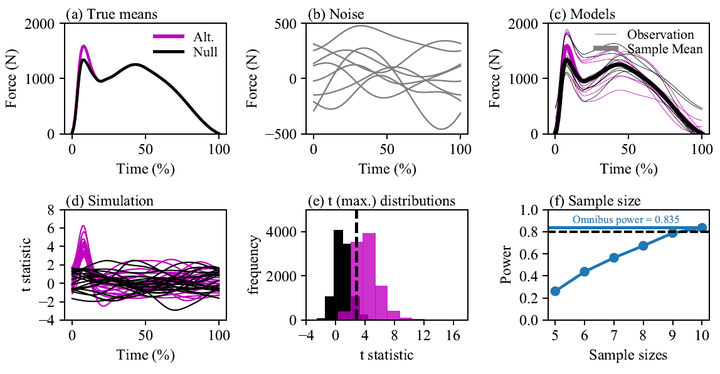# Sample size estimation for biomechanical waveforms: Current practice, recommendations and a comparison to discrete power analysis### Abstract

Testing a prediction is fundamental to scientific experiments. Where biomechanical experiments involve analysis of 1-Dimensional (waveform) data, sample size estimation should consider both 1D variance and hypothesised 1D effects. This study exemplifies 1D sample size estimation using typical biomechanical signals and contrasts this with 0D (discrete) power analysis. For context, biomechanics papers from 2018 and 2019 were reviewed to characterise current practice. Sample size estimation occurred in approximately 4% of 653 papers and reporting practice was mixed. To estimate sample sizes, common biomechanical signals were sourced from the literature and 1D effects were generated artificially using the open-source power1d software. Smooth Gaussian noise was added to the modelled 1D effect to numerically estimate the sample size required. Sample sizes estimated using 1D power procedures varied according to the characteristics of the dataset, requiring only small-to moderate sample sizes of approximately 5-40 to achieve target powers of 0.8 for reported 1D effects, but were always larger than 0D sample sizes (from N+1 to >N+20). The importance of a-priori sample size estimation is highlighted and recommendations are provided to improve the consistency of reporting. This study should enable researchers to construct 1D biomechanical effects to address adequately powered, hypothesis-driven, predictive research questions.

Publication
Journal of Biomechanics